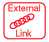# Kutta-Joukowski Lift TheoremTwo early aerodynamicists, Kutta in Germany and Joukowski in Russia, worked to quantify the lift achieved by an airflow over a spinning cylinder. The lift relationship is

Lift per unit length = L = ρGV

where ρ is the air density, V is the velocity of flow, and G is called the "vortex strength". The vortex strength is given by

G = 2ρωr2

where ω is the angular velocity of spin of the cylinder.

Like all aerodynamic lift, this seems a bit mysterious, but it can be looked at in terms of a redirection of the air motion. If the cylinder traps some air in a boundary layer at the cylinder surface and carries it around with it, shedding it downward, then it has given some of the air a downward momentum. That can act to give the cylinder an upward momentum in accordance with the principle of conservation of momentum. Another approach is to say that you have exerted a downward component of force on the air and by Newton's 3rd law there must be an upward force on the cylinder. Yet another approach is to say that the top of the cylinder is assisting the airstream, speeding up the flow on the top of the cylinder. Then by the Bernoulli equation, the pressure on the top of the cylinder is diminished, giving an effective lift.

Index

Bernoulli concepts

References
EastlakeNASA
Aerodynamics

 HyperPhysics***** Mechanics ***** Fluids R Nave
Go Back